# Dispersion interaction

In chemistry and physics, the dispersion interaction is one of the forces acting between stable molecules. The dispersion interaction operates between molecules and atoms (referred to as monomers) of any kind and is always attractive when the monomers are in their ground state. That is, dispersion pulls monomers together. Between noble gas atoms it is the only attractive intermolecular force and hence it is the cause of noble gases undergoing phase transitions to stable liquids and solids. (These phase transitions happen for low temperature and/or high pressure). Also for non-polar molecules such as methane, benzene, and other hydrocarbons, dispersion gives one of the most important attractive forces between the molecules. It explains, for instance, why benzene under standard temperature and pressure (STP) is a liquid.

In 1930 R. Eisenschitz and F. London gave the first complete explanation of intermolecular forces (also referred to as van der Waals forces). They distinguished different effects and among them the effect that we know now as dispersion interaction. The corresponding dispersion force (also known as London force) falls of as R−7, where R is the distance between the monomers. Eisenschitz and London point out that so-called oscillator strengths f are an important ingredient of their theoretical description. These f-values entered previously the classical theory of the dispersion of light formulated by Lorentz and Drude. The same f-values also appear in the "old" (Planck-Bohr-Sommerfeld) quantum theory of dispersion formulated by Kramers and Heisenberg. Because of the similarity between the several theories needing f-values, London baptized the corresponding part of the intermolecular interaction the dispersion effect.

The Eisenschitz-London work being very complete and mathematically sophisticated, London gave in the same year an easier description of the dispersion force in which he modeled each monomer of a dimer as a three-dimensional isotropic harmonic oscillator. The polarizable oscillators consist of a reduced particle of mass m and two charges e of opposite sign, i.e., each monomer is modeled as an oscillating electric dipole. The two dipoles interact through the electric Coulomb force. By an approximate quantum mechanical treatment London derived the following form of the interaction potential of the oscillators in their ground (lowest energy) state: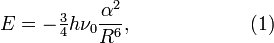$E = -\tfrac{3}{4} h\nu_0 \frac{\alpha^2}{R^6}, \qquad\qquad\qquad(1)$

an expression which is similar (quadratic in the polarizability α, depending on R−6) to the dispersion formula he derived together with Eisenschitz for real atoms and molecules. The quantity hν0 is the zero point energy of one oscillator. The gradient (derivative of E with respect to R) is proportional to R−7 and remember that minus the gradient is the attractive force associated with a potential. London stresses that his formula is due to the correlation between the zero point motions of the free oscillators. The zero point motion is a typical quantum mechanical phenomenon related to Heisenberg's uncertainty principle. Without zero point motion, London's model does not give an attraction between oscillators in their ground state. London also points out that the Planck-Bohr-Sommerfeld quantum theory does not account for the zero point motion of harmonic oscillators and accordingly cannot explain the dispersion attraction between two oscillators in their ground state.

In this model the totality of electrons in an atom is represented by one harmonically oscillating dipole. Representing atomic electrons by oscillators was a common procedure before the discovery of quantum mechanics: Lorentz explained the Zeeman effect by it, Planck assumed that a radiating black body consists of oscillators (which he assumed quantized), and also in the theory of Drude, Kramers, and Heisenberg for the dispersion of light, oscillating electrons ultimately explain the effects under study. This familiarity with the model is probably the reason that London did not attempt to explain why, for instance, two noble gas atoms would resemble quantum mechanical oscillators when they interact.

In the section London (dispersion) forces of the article intermolecular forces, the Eisenschitz-London theory, derived by perturbation theory and based on oscillator strengths, is discussed. In the present article the simple London model of two interacting oscillating dipoles will be worked out in some detail.

## Proof of equation (1)

The London theory leading to equation (1) will be reviewed. The theory is based on two interacting three-dimensional isotropic harmonic oscillators. Isotropy means that the spring (Hooke) constants are equal: kx = ky = kzk. An isotropic three-dimensional oscillator factorizes into three identical one-dimensional oscillators and the total energy of one three-dimensional oscillator is the sum of energies of three one-dimensional oscillators.

### Oscillating dipole

A one-dimensional electric dipole μ0 consists of two equal charges e of opposite sign a distance x0 apart, μ0 = e x0. In an external electric field E the distance between the charges will change and correspondingly the dipole does too:$\Delta \mu = e\Delta x = \alpha\; E$

where α is the polarizability of the system. Hooke's law states that there is the spring force kΔx, which balances the force eE by the external field$k\Delta x = e E\; \Longrightarrow\; k \Delta\mu = e^2 E \Longrightarrow\; k\alpha E = e^2 E$

so that Hooke's constant is seen to be inversely proportional to the polarizability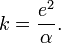$k = \frac{e^2}{\alpha}. \;$

### Quantum mechanical harmonic oscillator

The potential corresponding to the force kx is ½ k x2. Let the mass of the oscillating particle be m (this is the reduced mass of the two charged particles) and the Schrödinger equation becomes,$\left[-\frac{\hbar^2}{2m} \frac{d^2}{dx^2} + \frac{1}{2} kx^2\right] \Psi_n = \mathcal{E}_n\Psi_n,$

where ℏ is the reduced Planck constant. It is shown in the article on the quantum harmonic oscillator that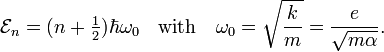$\mathcal{E}_n = (n+\tfrac{1}{2}) \hbar\omega_0\quad\hbox{with}\quad \omega_0 = \sqrt{\frac{k}{m}} = \frac{e}{\sqrt{m\alpha}}.$

### Interaction

One can expand the total Coulomb interaction in a series of interacting multipoles of the two monomers. Since electric dipoles are neutral, the first non-vanishing term in the expansion is the dipole-dipole interaction. London only considered this first term. If we place the z-axis along the vector R that connects the two dipoles, this interaction takes the form$\frac{1}{R^3} \left(\boldsymbol{\mu}_1\cdot\boldsymbol{\mu}_2 - 3\frac{(\boldsymbol{\mu}_1\cdot\mathbf{R})\,(\boldsymbol{\mu}_2\cdot\mathbf{R})}{R^2} \right)= \frac{e^2}{R^3}\left( x_1x_2 + y_1y_2 -2 z_1z_2\right),$

here R = |R| and the indices 1 and 2 label the monomers.

### Schrödinger equation of dimer

The total 6-dimensional Schrödinger equation becomes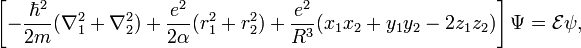$\left[- \frac{\hbar^2}{2m} (\nabla_1^2 + \nabla_2^2) + \frac{e^2}{2\alpha}(r_1^2+r_2^2) +\frac{e^2}{R^3}( x_1x_2 + y_1y_2 -2 z_1z_2)\right] \Psi = \mathcal{E} \psi,$

where r stands for |r| and is the nabla operator consisting of derivatives with respect to x, y, and z.

### Solution

We make the following substitutions to obtain a 6-dimensional Schrödinger equation that can be factorized into 6 one-dimensional Schrödinger equations of the harmonic oscillator type,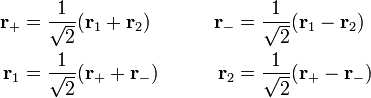\begin{align} \mathbf{r}_{+} &= \frac{1}{\sqrt{2}}(\mathbf{r}_{1} + \mathbf{r}_{2}) &\qquad \mathbf{r}_{-} &= \frac{1}{\sqrt{2}}(\mathbf{r}_{1} - \mathbf{r}_{2})\\ \mathbf{r}_{1} &= \frac{1}{\sqrt{2}}(\mathbf{r}_{+} + \mathbf{r}_{-}) &\qquad \mathbf{r}_{2} &= \frac{1}{\sqrt{2}}(\mathbf{r}_{+} - \mathbf{r}_{-}) \end{align}

The Schrödinger equation becomes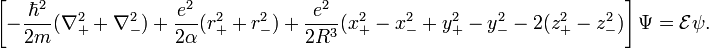$\left[- \frac{\hbar^2}{2m} (\nabla_{+}^2 + \nabla_{-}^2) + \frac{e^2}{2\alpha}(r_{+}^2+r_{-}^2) +\frac{e^2}{2R^3}( x_{+}^2 - x_{-}^2 + y_{+}^2 - y_{-}^2 -2(z_{+}^2 - z_{-}^2 )\right] \Psi = \mathcal{E} \psi.$

It factorizes into the following six equations\begin{align} \left[ -\frac{\hbar^2}{2m} \frac{d^2}{dx_{+}^2} + x_{+}^2 \Big(\frac{1}{\alpha}+\frac{1}{R^3}\Big) \frac{e^2}{2} \right] \psi_{n^{+}_x} &= \mathcal{E}^{+}_x \psi_{n^{+}_x} \\ \left[ -\frac{\hbar^2}{2m} \frac{d^2}{dy_{+}^2} + y_{+}^2 \Big(\frac{1}{\alpha}+\frac{1}{R^3}\Big) \frac{e^2}{2} \right] \psi_{n^{+}_y} &= \mathcal{E}^{+}_y \psi_{n^{+}_y} \\ \left[ -\frac{\hbar^2}{2m} \frac{d^2}{dx_{-}^2} + x_{-}^2 \Big(\frac{1}{\alpha}-\frac{1}{R^3}\Big) \frac{e^2}{2} \right] \psi_{n^{-}_x} &= \mathcal{E}^{-}_x \psi_{n^{-}_x} \\ \left[ -\frac{\hbar^2}{2m} \frac{d^2}{dy_{-}^2} + y_{-}^2 \Big(\frac{1}{\alpha}-\frac{1}{R^3}\Big) \frac{e^2}{2} \right] \psi_{n^{-}_y} &= \mathcal{E}^{-}_y \psi_{n^{-}_y} \\ \left[ -\frac{\hbar^2}{2m} \frac{d^2}{dz_{+}^2} + z_{+}^2 \Big(\frac{1}{\alpha}-\frac{2}{R^3}\Big) \frac{e^2}{2} \right] \psi_{n^{+}_z} &= \mathcal{E}^{+}_z \psi_{n^{+}_z} \\ \left[ -\frac{\hbar^2}{2m} \frac{d^2}{dz_{-}^2} + z_{-}^2 \Big(\frac{1}{\alpha}+\frac{2}{R^3}\Big) \frac{e^2}{2} \right] \psi_{n^{-}_z} &= \mathcal{E}^{-}_z \psi_{n^{-}_z} \\ \end{align}

The total energy is the sum$\mathcal{E} = \mathcal{E}^{+}_x + \mathcal{E}^{+}_y + \mathcal{E}^{-}_x + \mathcal{E}^{-}_y + \mathcal{E}^{+}_z + \mathcal{E}^{-}_z.$

The 6 equations are are one-dimensional harmonic oscillator equations with the following ω values:\begin{align} \omega^{+}_x = \omega_0 \sqrt{1 + \frac{\alpha}{R^3}} &&\qquad \omega^{-}_x = \omega_0 \sqrt{1 - \frac{\alpha}{R^3}} &&\qquad \omega^{+}_y = \omega_0 \sqrt{1 + \frac{\alpha}{R^3}} &&\\ \omega^{-}_y = \omega_0 \sqrt{1 - \frac{\alpha}{R^3}} &&\qquad \omega^{+}_z = \omega_0 \sqrt{1 - \frac{2\alpha}{R^3}} &&\qquad \omega^{-}_z = \omega_0 \sqrt{1 + \frac{2\alpha}{R^3}}, &&\\ \end{align}

where ω0 = e/(mα)½ was introduced earlier. The exact energy is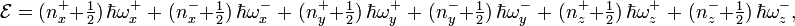$\mathcal{E} = (n^{+}_x + \tfrac{1}{2})\,\hbar\omega^{+}_x \;+\; (n^{-}_x + \tfrac{1}{2})\,\hbar\omega^{-}_x \;+\; (n^{+}_y + \tfrac{1}{2})\,\hbar\omega^{+}_y \;+\; (n^{-}_y + \tfrac{1}{2})\,\hbar\omega^{-}_y \;+\; (n^{+}_z + \tfrac{1}{2})\,\hbar\omega^{+}_z \;+\; (n^{-}_z + \tfrac{1}{2})\,\hbar\omega^{-}_z ,$

where the six n-quantum numbers run independently over 0, 1, 2, ….

### Expansion

We now make an expansion of the exact energy that is the analogue of the perturbation expansion and use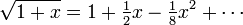$\sqrt{1+x} = 1 + \tfrac{1}{2} x - \tfrac{1}{8} x^2 + \cdots$

in the expansion of the six ω values. Collecting terms multiplying the same power of R, we get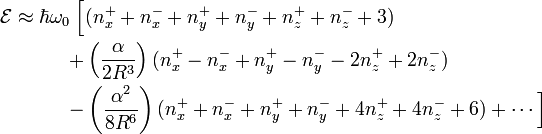\begin{align} \mathcal{E} \approx \hbar\omega_0\; \Big[&( n_x^{+} + n_x^{-} + n_y^{+} + n_y^{-} + n_z^{+} + n_z^{-} + 3) \\ +&\left(\frac{\alpha}{2R^3}\right) ( n_x^{+} - n_x^{-} + n_y^{+} - n_y^{-} -2 n_z^{+} +2 n_z^{-}) \\ -&\left(\frac{\alpha^2}{8R^6}\right) ( n_x^{+} + n_x^{-} + n_y^{+} + n_y^{-} + 4 n_z^{+} +4 n_z^{-} +6) +\cdots \Big] \\ \end{align}

The first term gives the energy of the two free (i.e., non-interacting) oscillators. In a perturbation theory approach this would be the zeroth-order term. The second term vanishes if the system is in its ground state (all 6 n-quantum numbers equal to zero). In a perturbation approach it would be a first-order resonance energy when one monomer is in its ground state and the other in an excited state and the excitation "jumps back and forth between the monomers" (in the quantum mechanical sense). The third term is the dispersion term. Noting that ℏω0 = hν0, it is the energy in equation (1) of the introduction when all 6 n-quantum numbers are equal to zero.

Observe that the number 6 in the third term arises from the zero point energies of the one-dimensional harmonic oscillators. In the "old" quantum theory the energy of a harmonic oscillator is n ℏ ω0, instead of (n+½) ℏ ω0, so that in the old quantum theory the third term vanishes for a system in its ground state. Since the zero point motion of a harmonic oscillator is due to the typical quantum mechanical effect that x cannot be not sharp—does not have one well-defined value—when the state of the system is an energy eigenstate, it can be concluded that London's oscillator model shows that dispersion is a quantum mechanical effect.